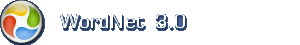# Engelsk ordbog

Tip: Spørgsmålstegn (?) kan anvendes som jokertegn (wild card). Spørgsmålstegnet erstatter præcis et tegn.

## Engelsk navneord: quantity

1. quantity how much there is or how many there are of something that you can quantify

Termer med samme betydning (synonymer)amount, measure

Mindre specifikke termerabstract entity, abstraction

Mere specifikke termerchance, cordage, definite quantity, economic value, fundamental measure, fundamental quantity, indefinite quantity, interval, magnetisation, magnetization, metric, octane number, octane rating, period of play, play, playing period, point, point in time, probability, proof, quantum, radical, relative quantity, system of measurement, time interval, time unit, unit of time, value, volume, volume

Kendetegnernonstandard, standard

2. quantity (om egenskab) an adequate or large amount

Eksempler med tilsvarende betydningHe had a quantity of ammunition.

Mindre specifikke termeramount

Mere specifikke termerabundance, copiousness, teemingness

Kendetegnerabundant, deficient, insufficient, scarce, sufficient

3. quantity (om erkendelse) the concept that something has a magnitude and can be represented in mathematical expressions by a constant or a variable

Mindre specifikke termerconcept, conception, construct

Mere specifikke termeramount, binomial, constant, constant quantity, invariable, mathematical product, numerical quantity, operand, parameter, product, proportional, quantum, sum, term, total, variable, variable quantity